oomph::PseudoBucklingRingElement Class Reference

//////////////////////////////////////////////////////////////////// More...

#include <pseudo_buckling_ring.h>Inheritance diagram for oomph::PseudoBucklingRingElement:

## Public Member Functions

PseudoBucklingRingElement (const double &eps_buckl, const double &ampl_ratio, const unsigned n_buckl, const double &r_0, const double &T, TimeStepper *time_stepper_pt)
Constructor: Build pseudo buckling ring from doubles that describe the geometry. More...

PseudoBucklingRingElement (const double &eps_buckl, const double &HoR, const unsigned &n_buckl, const unsigned &imode, TimeStepper *time_stepper_pt)
Constructor: Pass buckling amplitude, h/R, buckling wavenumbe and pointer to global timestepper. Other parameters get set up to represent oscillating ring with mode imode (1 or 2). All geometric data is pinned by default. More...

PseudoBucklingRingElement (const PseudoBucklingRingElement &dummy)=delete
Broken copy constructor. More...

void operator= (const PseudoBucklingRingElement &)=delete
Broken assignment operator. More...

virtual ~PseudoBucklingRingElement ()
Destructor: Kill internal data and set to NULL. More...

virtual void get_residuals (Vector< double > &residuals)
Compute element residual Vector (wrapper) More...

virtual void get_jacobian (Vector< double > &residuals, DenseMatrix< double > &jacobian)
Compute element residual Vector and element Jacobian matrix (wrapper) More...

Data *const & reference_pressure_pt () const
Pointer to pressure data that is used as reference pressure. More...

double reference_pressure () const
Return the reference pressure. More...

void set_reference_pressure_pt (Data *const &data_pt)
Set the pressure data that is used as reference pressure. More...Public Member Functions inherited from oomph::GeneralisedElement
GeneralisedElement() GeneralisedElement (const GeneralisedElement &)=delete
Constructor: Initialise all pointers and all values to zero. More...

void operator= (const GeneralisedElement &)=delete
Broken assignment operator. More...

Data *& internal_data_pt (const unsigned &i)
Return a pointer to i-th internal data object. More...

Data *const & internal_data_pt (const unsigned &i) const
Return a pointer to i-th internal data object (const version) More...

Data *& external_data_pt (const unsigned &i)
Return a pointer to i-th external data object. More...

Data *const & external_data_pt (const unsigned &i) const
Return a pointer to i-th external data object (const version) More...

unsigned long eqn_number (const unsigned &ieqn_local) const
Return the global equation number corresponding to the ieqn_local-th local equation number. More...

int local_eqn_number (const unsigned long &ieqn_global) const
Return the local equation number corresponding to the ieqn_global-th global equation number. Returns minus one (-1) if there is no local degree of freedom corresponding to the chosen global equation number. More...

unsigned add_external_data (Data *const &data_pt, const bool &fd=true)
Add a (pointer to an) external data object to the element and return its index (i.e. the index required to obtain it from the access function external_data_pt(...). The optional boolean flag indicates whether the data should be included in the general finite-difference loop when calculating the jacobian. The default value is true, i.e. the data will be included in the finite-differencing. More...

bool external_data_fd (const unsigned &i) const
Return the status of the boolean flag indicating whether the external data is included in the finite difference loop. More...

void exclude_external_data_fd (const unsigned &i)
Set the boolean flag to exclude the external datum from the the finite difference loop when computing the jacobian matrix. More...

void include_external_data_fd (const unsigned &i)
Set the boolean flag to include the external datum in the the finite difference loop when computing the jacobian matrix. More...

void flush_external_data ()
Flush all external data. More...

void flush_external_data (Data *const &data_pt)
Flush the object addressed by data_pt from the external data array. More...

unsigned ninternal_data () const
Return the number of internal data objects. More...

unsigned nexternal_data () const
Return the number of external data objects. More...

unsigned ndof () const
Return the number of equations/dofs in the element. More...

void dof_vector (const unsigned &t, Vector< double > &dof)
Return the vector of dof values at time level t. More...

void dof_pt_vector (Vector< double * > &dof_pt)
Return the vector of pointers to dof values. More...

void set_internal_data_time_stepper (const unsigned &i, TimeStepper *const &time_stepper_pt, const bool &preserve_existing_data)
Set the timestepper associated with the i-th internal data object. More...

void assign_internal_eqn_numbers (unsigned long &global_number, Vector< double * > &Dof_pt)
Assign the global equation numbers to the internal Data. The arguments are the current highest global equation number (which will be incremented) and a Vector of pointers to the global variables (to which any unpinned values in the internal Data are added). More...

void describe_dofs (std::ostream &out, const std::string &current_string) const
Function to describe the dofs of the element. The ostream specifies the output stream to which the description is written; the string stores the currently assembled output that is ultimately written to the output stream by Data::describe_dofs(...); it is typically built up incrementally as we descend through the call hierarchy of this function when called from Problem::describe_dofs(...) More...

virtual void describe_local_dofs (std::ostream &out, const std::string &current_string) const
Function to describe the local dofs of the element. The ostream specifies the output stream to which the description is written; the string stores the currently assembled output that is ultimately written to the output stream by Data::describe_dofs(...); it is typically built up incrementally as we descend through the call hierarchy of this function when called from Problem::describe_dofs(...) More...

void add_internal_value_pt_to_map (std::map< unsigned, double * > &map_of_value_pt)
Add pointers to the internal data values to map indexed by the global equation number. More...

void add_internal_data_values_to_vector (Vector< double > &vector_of_values)
Add all internal data and time history values to the vector in the internal storage order. More...

void read_internal_data_values_from_vector (const Vector< double > &vector_of_values, unsigned &index)
Read all internal data and time history values from the vector starting from index. On return the index will be set to the value at the end of the data that has been read in. More...

void add_internal_eqn_numbers_to_vector (Vector< long > &vector_of_eqn_numbers)
Add all equation numbers associated with internal data to the vector in the internal storage order. More...

void read_internal_eqn_numbers_from_vector (const Vector< long > &vector_of_eqn_numbers, unsigned &index)
Read all equation numbers associated with internal data from the vector starting from index. On return the index will be set to the value at the end of the data that has been read in. More...

virtual void assign_local_eqn_numbers (const bool &store_local_dof_pt)
Setup the arrays of local equation numbers for the element. If the optional boolean argument is true, then pointers to the associated degrees of freedom are stored locally in the array Dof_pt. More...

virtual void complete_setup_of_dependencies ()
Complete the setup of any additional dependencies that the element may have. Empty virtual function that may be overloaded for specific derived elements. Used, e.g., for elements with algebraic node update functions to determine the "geometric Data", i.e. the Data that affects the element's shape. This function is called (for all elements) at the very beginning of the equation numbering procedure to ensure that all dependencies are accounted for. More...

virtual void get_mass_matrix (Vector< double > &residuals, DenseMatrix< double > &mass_matrix)
Calculate the residuals and the elemental "mass" matrix, the matrix that multiplies the time derivative terms in a problem. More...

virtual void get_jacobian_and_mass_matrix (Vector< double > &residuals, DenseMatrix< double > &jacobian, DenseMatrix< double > &mass_matrix)
Calculate the residuals and jacobian and elemental "mass" matrix, the matrix that multiplies the time derivative terms. More...

virtual void get_dresiduals_dparameter (double *const &parameter_pt, Vector< double > &dres_dparam)
Calculate the derivatives of the residuals with respect to a parameter. More...

virtual void get_djacobian_dparameter (double *const &parameter_pt, Vector< double > &dres_dparam, DenseMatrix< double > &djac_dparam)
Calculate the derivatives of the elemental Jacobian matrix and residuals with respect to a parameter. More...

virtual void get_djacobian_and_dmass_matrix_dparameter (double *const &parameter_pt, Vector< double > &dres_dparam, DenseMatrix< double > &djac_dparam, DenseMatrix< double > &dmass_matrix_dparam)
Calculate the derivatives of the elemental Jacobian matrix mass matrix and residuals with respect to a parameter. More...

virtual void get_hessian_vector_products (Vector< double > const &Y, DenseMatrix< double > const &C, DenseMatrix< double > &product)
Calculate the product of the Hessian (derivative of Jacobian with respect to all variables) an eigenvector, Y, and other specified vectors, C (d(J_{ij})/d u_{k}) Y_{j} C_{k}. More...

virtual void get_inner_products (Vector< std::pair< unsigned, unsigned > > const &history_index, Vector< double > &inner_product)
Return the vector of inner product of the given pairs of history values. More...

virtual void get_inner_product_vectors (Vector< unsigned > const &history_index, Vector< Vector< double > > &inner_product_vector)
Compute the vectors that when taken as a dot product with other history values give the inner product over the element. More...

virtual unsigned self_test ()
Self-test: Have all internal values been classified as pinned/unpinned? Return 0 if OK. More...

virtual void compute_norm (Vector< double > &norm)
Compute norm of solution – broken virtual can be overloaded by element writer to implement whatever norm is desired for the specific element. More...

virtual void compute_norm (double &norm)
Compute norm of solution – broken virtual can be overloaded by element writer to implement whatever norm is desired for the specific element. More...

void set_halo (const unsigned &non_halo_proc_ID)
Label the element as halo and specify processor that holds non-halo counterpart. More...

void set_nonhalo ()
Label the element as not being a halo. More...

bool is_halo () const
Is this element a halo? More...

int non_halo_proc_ID ()
ID of processor ID that holds non-halo counterpart of halo element; negative if not a halo. More...

void set_must_be_kept_as_halo ()
Insist that this element be kept as a halo element during a distribute? More...

void unset_must_be_kept_as_halo ()
Do not insist that this element be kept as a halo element during distribution. More...

bool must_be_kept_as_halo () const
Test whether the element must be kept as a halo element. More...

virtual unsigned ndof_types () const
The number of types of degrees of freedom in this element are sub-divided into. More...

virtual void get_dof_numbers_for_unknowns (std::list< std::pair< unsigned long, unsigned > > &dof_lookup_list) const
Create a list of pairs for the unknowns that this element is "in charge of" – ignore any unknowns associated with external Data. The first entry in each pair must contain the global equation number of the unknown, while the second one contains the number of the DOF type that this unknown is associated with. (The function can obviously only be called if the equation numbering scheme has been set up.) More...Public Member Functions inherited from oomph::PseudoBucklingRing
PseudoBucklingRing ()
Default constructor (empty and broken) More...

PseudoBucklingRing (const Vector< Data * > &geom_data_pt, TimeStepper *time_stepper_pt)
Constructor: 1 Lagrangian coordinate, 2 Eulerian coords. Pass buckling amplitude, ratio of of buckling amplitudes, buckling wavenumber (as a double), undeformed ring radius (all as Data) and pointer to global timestepper. More...

PseudoBucklingRing (const double &eps_buckl, const double &ampl_ratio, const unsigned n_buckl, const double &r_0, const double &T, TimeStepper *time_stepper_pt)
Constructor: 1 Lagrangian coordinate, 2 Eulerian coords. Pass buckling amplitude, ratio of of buckling amplitudes, buckling wavenumber, undeformed ring radius, period of osc and pointer to global timestepper. All geometric data is pinned by default. More...

PseudoBucklingRing (const double &eps_buckl, const double &HoR, const unsigned &n_buckl, const unsigned &imode, TimeStepper *time_stepper_pt)
Constructor: 1 Lagrangian coordinate, 2 Eulerian coords. Pass buckling amplitude, h/R, buckling wavenumbe and pointer to global timestepper. Other parameters get set up to represent oscillating ring with mode imode (1 or 2). All geometric data is pinned by default. More...

PseudoBucklingRing (const PseudoBucklingRing &node)=delete
Broken copy constructor. More...

void operator= (const PseudoBucklingRing &)=delete
Broken assignment operator. More...

~PseudoBucklingRing ()
Destructor: Clean up if necessary. More...

double eps_buckl ()
Access function for buckling amplitude. More...

double ampl_ratio ()
Access function for amplitude ratio. More...

double r_0 ()
Access function for undeformed radius. More...

double T ()
Access function for period of oscillation. More...

double n_buckl_float ()
Access function for buckling wavenumber (as float) More...

void set_eps_buckl (const double &eps_buckl)
Set buckling amplitude. More...

void set_ampl_ratio (const double &ampl_ratio)
Set amplitude ratio between radial and azimuthal buckling displacements. More...

void set_n_buckl (const unsigned &n_buckl)
Set buckling wavenumber. More...

void set_R_0 (const double &r_0)
Set undeformed radius of ring. More...

void set_T (const double &T)
Set period of oscillation. More...

void position (const Vector< double > &zeta, Vector< double > &r) const
Position Vector at Lagrangian coordinate zeta at present time. More...

void veloc (const Vector< double > &zeta, Vector< double > &veloc)
Parametrised velocity on object at current time: veloc = d r(zeta)/dt. More...

void accel (const Vector< double > &zeta, Vector< double > &accel)
Parametrised acceleration on object at current time: accel = d^2 r(zeta)/dt^2. More...

void position (const unsigned &t, const Vector< double > &zeta, Vector< double > &r) const
Position Vector at Lagrangian coordinate zeta at discrete previous time (t=0: present time; t>0: previous time) More...

void dposition_dt (const Vector< double > &zeta, const unsigned &j, Vector< double > &drdt)
j-th time-derivative on object at current time: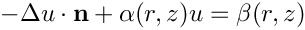. More...

unsigned ngeom_data () const
How many items of Data does the shape of the object depend on? More...

Datageom_data_pt (const unsigned &j)
Return pointer to the j-th Data item that the object's shape depends on. More...Public Member Functions inherited from oomph::GeomObject
GeomObject ()
Default constructor. More...

GeomObject (const unsigned &ndim)
Constructor: Pass dimension of geometric object (# of Eulerian coords = # of Lagrangian coords; no time history available/needed) More...

GeomObject (const unsigned &nlagrangian, const unsigned &ndim)
Constructor: pass # of Eulerian and Lagrangian coordinates. No time history available/needed. More...

GeomObject (const unsigned &nlagrangian, const unsigned &ndim, TimeStepper *time_stepper_pt)
Constructor: pass # of Eulerian and Lagrangian coordinates and pointer to time-stepper which is used to handle the position at previous timesteps and allows the evaluation of veloc/acceleration etc. in cases where the GeomData varies with time. More...

GeomObject (const GeomObject &dummy)=delete
Broken copy constructor. More...

void operator= (const GeomObject &)=delete
Broken assignment operator. More...

virtual ~GeomObject ()
(Empty) destructor More...

unsigned nlagrangian () const
Access function to # of Lagrangian coordinates. More...

unsigned ndim () const
Access function to # of Eulerian coordinates. More...

void set_nlagrangian_and_ndim (const unsigned &n_lagrangian, const unsigned &n_dim)
Set # of Lagrangian and Eulerian coordinates. More...

TimeStepper *& time_stepper_pt ()
Access function for pointer to time stepper: Null if object is not time-dependent. More...

TimeSteppertime_stepper_pt () const
Access function for pointer to time stepper: Null if object is not time-dependent. Const version. More...

virtual void position (const double &t, const Vector< double > &zeta, Vector< double > &r) const
Parametrised position on object: r(zeta). Evaluated at the continuous time value, t. More...

virtual void dposition (const Vector< double > &zeta, DenseMatrix< double > &drdzeta) const
Derivative of position Vector w.r.t. to coordinates: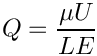= drdzeta(alpha,i). Evaluated at current time. More...

virtual void d2position (const Vector< double > &zeta, RankThreeTensor< double > &ddrdzeta) const
2nd derivative of position Vector w.r.t. to coordinates: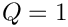= ddrdzeta(alpha,beta,i). Evaluated at current time. More...

virtual void d2position (const Vector< double > &zeta, Vector< double > &r, DenseMatrix< double > &drdzeta, RankThreeTensor< double > &ddrdzeta) const
Posn Vector and its 1st & 2nd derivatives w.r.t. to coordinates:= drdzeta(alpha,i).= ddrdzeta(alpha,beta,i). Evaluated at current time. More...

virtual void locate_zeta (const Vector< double > &zeta, GeomObject *&sub_geom_object_pt, Vector< double > &s, const bool &use_coordinate_as_initial_guess=false)
A geometric object may be composed of may sub-objects (e.g. a finite-element representation of a boundary). In order to implement sparse update functions, it is necessary to know the sub-object and local coordinate within that sub-object at a given intrinsic coordinate, zeta. Note that only one sub-object can "cover" any given intrinsic position. If the position is at an "interface" between sub-objects, either one can be returned. The default implementation merely returns, the pointer to the "entire" GeomObject and the coordinate, zeta The optional boolean flag only applies if a Newton method is used to find the value of zeta, and if true the value of the coordinate s is used as the initial guess for the method. If the flag is false (the default) a value of s=0 is used as the initial guess. More...

virtual void interpolated_zeta (const Vector< double > &s, Vector< double > &zeta) const
A geometric object may be composed of many sub-objects each with their own local coordinate. This function returns the "global" intrinsic coordinate zeta (within the compound object), at a given local coordinate s (i.e. the intrinsic coordinate of the sub-GeomObject. In simple (non-compound) GeomObjects, the local intrinsic coordinate is the global intrinsic coordinate and so the function merely returns s. To make it less likely that the default implementation is called in error (because it is not overloaded in a derived GeomObject where the default is not appropriate, we do at least check that s and zeta have the same size if called in PARANOID mode. More...

## Protected Member Functions

virtual void get_residuals_generic (Vector< double > &residuals, DenseMatrix< double > &jacobian, unsigned flag)
Compute element residual Vector (only if flag=0) and also element Jacobian matrix (if flag=1) More...Protected Member Functions inherited from oomph::GeneralisedElement
unsigned add_internal_data (Data *const &data_pt, const bool &fd=true)
Add a (pointer to an) internal data object to the element and return the index required to obtain it from the access function internal_data_pt(). The boolean indicates whether the datum should be included in the general finite-difference loop when calculating the jacobian. The default value is true, i.e. the data will be included in the finite differencing. More...

bool internal_data_fd (const unsigned &i) const
Return the status of the boolean flag indicating whether the internal data is included in the finite difference loop. More...

void exclude_internal_data_fd (const unsigned &i)
Set the boolean flag to exclude the internal datum from the finite difference loop when computing the jacobian matrix. More...

void include_internal_data_fd (const unsigned &i)
Set the boolean flag to include the internal datum in the finite difference loop when computing the jacobian matrix. More...

void clear_global_eqn_numbers ()
Clear the storage for the global equation numbers and pointers to dofs (if stored) More...

void add_global_eqn_numbers (std::deque< unsigned long > const &global_eqn_numbers, std::deque< double * > const &global_dof_pt)
Add the contents of the queue global_eqn_numbers to the local storage for the local-to-global translation scheme. It is essential that the entries in the queue are added IN ORDER i.e. from the front. More...

virtual void assign_internal_and_external_local_eqn_numbers (const bool &store_local_dof_pt)
Assign the local equation numbers for the internal and external Data This must be called after the global equation numbers have all been assigned. It is virtual so that it can be overloaded by ElementWithExternalElements so that any external data from the external elements in included in the numbering scheme. If the boolean argument is true then pointers to the dofs will be stored in Dof_pt. More...

virtual void assign_all_generic_local_eqn_numbers (const bool &store_local_dof_pt)
Assign all the local equation numbering schemes that can be applied generically for the element. In most cases, this is the function that will be overloaded by inherited classes. It is required to ensure that assign_additional_local_eqn_numbers() can always be called after ALL other local equation numbering has been performed. The default for the GeneralisedElement is simply to call internal and external local equation numbering. If the boolean argument is true then pointers to the dofs will be stored in Dof_pt. More...

Setup any additional look-up schemes for local equation numbers. Examples of use include using local storage to refer to explicit degrees of freedom. The additional memory cost of such storage may or may not be offset by fast local access. More...

int internal_local_eqn (const unsigned &i, const unsigned &j) const
Return the local equation number corresponding to the j-th value stored at the i-th internal data. More...

int external_local_eqn (const unsigned &i, const unsigned &j)
Return the local equation number corresponding to the j-th value stored at the i-th external data. More...

virtual void fill_in_contribution_to_residuals (Vector< double > &residuals)
Add the elemental contribution to the residuals vector. Note that this function will NOT initialise the residuals vector. It must be called after the residuals vector has been initialised to zero. More...

void fill_in_jacobian_from_internal_by_fd (Vector< double > &residuals, DenseMatrix< double > &jacobian, const bool &fd_all_data=false)
Calculate the contributions to the jacobian from the internal degrees of freedom using finite differences. This version of the function assumes that the residuals vector has already been calculated. If the boolean argument is true, the finite differencing will be performed for all internal data, irrespective of the information in Data_fd. The default value (false) uses the information in Data_fd to selectively difference only certain data. More...

void fill_in_jacobian_from_internal_by_fd (DenseMatrix< double > &jacobian, const bool &fd_all_data=false)
Calculate the contributions to the jacobian from the internal degrees of freedom using finite differences. This version computes the residuals vector before calculating the jacobian terms. If the boolean argument is true, the finite differencing will be performed for all internal data, irrespective of the information in Data_fd. The default value (false) uses the information in Data_fd to selectively difference only certain data. More...

void fill_in_jacobian_from_external_by_fd (Vector< double > &residuals, DenseMatrix< double > &jacobian, const bool &fd_all_data=false)
Calculate the contributions to the jacobian from the external degrees of freedom using finite differences. This version of the function assumes that the residuals vector has already been calculated. If the boolean argument is true, the finite differencing will be performed for all external data, irrespective of the information in Data_fd. The default value (false) uses the information in Data_fd to selectively difference only certain data. More...

void fill_in_jacobian_from_external_by_fd (DenseMatrix< double > &jacobian, const bool &fd_all_data=false)
Calculate the contributions to the jacobian from the external degrees of freedom using finite differences. This version computes the residuals vector before calculating the jacobian terms. If the boolean argument is true, the finite differencing will be performed for all internal data, irrespective of the information in Data_fd. The default value (false) uses the information in Data_fd to selectively difference only certain data. More...

virtual void update_before_internal_fd ()
Function that is called before the finite differencing of any internal data. This may be overloaded to update any dependent data before finite differencing takes place. More...

virtual void reset_after_internal_fd ()
Function that is call after the finite differencing of the internal data. This may be overloaded to reset any dependent variables that may have changed during the finite differencing. More...

virtual void update_in_internal_fd (const unsigned &i)
Function called within the finite difference loop for internal data after a change in any values in the i-th internal data object. More...

virtual void reset_in_internal_fd (const unsigned &i)
Function called within the finite difference loop for internal data after the values in the i-th external data object are reset. The default behaviour is to call the update function. More...

virtual void update_before_external_fd ()
Function that is called before the finite differencing of any external data. This may be overloaded to update any dependent data before finite differencing takes place. More...

virtual void reset_after_external_fd ()
Function that is call after the finite differencing of the external data. This may be overloaded to reset any dependent variables that may have changed during the finite differencing. More...

virtual void update_in_external_fd (const unsigned &i)
Function called within the finite difference loop for external data after a change in any values in the i-th external data object. More...

virtual void reset_in_external_fd (const unsigned &i)
Function called within the finite difference loop for external data after the values in the i-th external data object are reset. The default behaviour is to call the update function. More...

virtual void fill_in_contribution_to_jacobian (Vector< double > &residuals, DenseMatrix< double > &jacobian)
Add the elemental contribution to the jacobian matrix. and the residuals vector. Note that this function will NOT initialise the residuals vector or the jacobian matrix. It must be called after the residuals vector and jacobian matrix have been initialised to zero. The default is to use finite differences to calculate the jacobian. More...

virtual void fill_in_contribution_to_mass_matrix (Vector< double > &residuals, DenseMatrix< double > &mass_matrix)
Add the elemental contribution to the mass matrix matrix. and the residuals vector. Note that this function should NOT initialise the residuals vector or the mass matrix. It must be called after the residuals vector and jacobian matrix have been initialised to zero. The default is deliberately broken. More...

virtual void fill_in_contribution_to_jacobian_and_mass_matrix (Vector< double > &residuals, DenseMatrix< double > &jacobian, DenseMatrix< double > &mass_matrix)
Add the elemental contribution to the jacobian matrix, mass matrix and the residuals vector. Note that this function should NOT initialise any entries. It must be called after the residuals vector and matrices have been initialised to zero. More...

virtual void fill_in_contribution_to_dresiduals_dparameter (double *const &parameter_pt, Vector< double > &dres_dparam)
Add the elemental contribution to the derivatives of the residuals with respect to a parameter. This function should NOT initialise any entries and must be called after the entries have been initialised to zero The default implementation is to use finite differences to calculate the derivatives. More...

virtual void fill_in_contribution_to_djacobian_dparameter (double *const &parameter_pt, Vector< double > &dres_dparam, DenseMatrix< double > &djac_dparam)
Add the elemental contribution to the derivatives of the elemental Jacobian matrix and residuals with respect to a parameter. This function should NOT initialise any entries and must be called after the entries have been initialised to zero The default implementation is to use finite differences to calculate the derivatives. More...

virtual void fill_in_contribution_to_djacobian_and_dmass_matrix_dparameter (double *const &parameter_pt, Vector< double > &dres_dparam, DenseMatrix< double > &djac_dparam, DenseMatrix< double > &dmass_matrix_dparam)
Add the elemental contribution to the derivative of the jacobian matrix, mass matrix and the residuals vector with respect to the passed parameter. Note that this function should NOT initialise any entries. It must be called after the residuals vector and matrices have been initialised to zero. More...

virtual void fill_in_contribution_to_hessian_vector_products (Vector< double > const &Y, DenseMatrix< double > const &C, DenseMatrix< double > &product)
Fill in contribution to the product of the Hessian (derivative of Jacobian with respect to all variables) an eigenvector, Y, and other specified vectors, C (d(J_{ij})/d u_{k}) Y_{j} C_{k}. More...

virtual void fill_in_contribution_to_inner_products (Vector< std::pair< unsigned, unsigned > > const &history_index, Vector< double > &inner_product)
Fill in the contribution to the inner products between given pairs of history values. More...

virtual void fill_in_contribution_to_inner_product_vectors (Vector< unsigned > const &history_index, Vector< Vector< double > > &inner_product_vector)
Fill in the contributions to the vectors that when taken as dot product with other history values give the inner product over the element. More...

## Private Member Functions

int geometric_local_eqn ()
Return the local equation number of the internal geometric variable. More...

int reference_pressure_local_eqn ()
Return the local equation number of the reference pressure variable. More...

## Private Attributes

unsigned Internal_geometric_variable_index
Index of the value stored in the single geometric object that has become an unknown. More...

unsigned External_reference_pressure_index
The Data object that represents the reference pressure is stored at the location indexed by this integer in the external data storage. More...

DataExternal_reference_pressure_pt
Pointer to the data object that represents the external reference pressure. More...Static Public Attributes inherited from oomph::GeneralisedElement
Static boolean to suppress warnings about repeated internal data. Defaults to false. More...

Static boolean to suppress warnings about repeated external data. Defaults to true. More...

static double Default_fd_jacobian_step = 1.0e-8
Double used for the default finite difference step in elemental jacobian calculations. More...Protected Attributes inherited from oomph::GeneralisedElement
int Non_halo_proc_ID
Non-halo processor ID for Data; -1 if it's not a halo. More...

bool Must_be_kept_as_halo
Does this element need to be kept as a halo element during a distribute? More...Protected Attributes inherited from oomph::PseudoBucklingRing
Vector< Data * > Geom_data_pt
Vector of pointers to Data items that affects the object's shape. More...

bool Must_clean_up
Do I need to clean up? More...Protected Attributes inherited from oomph::GeomObject
unsigned NLagrangian
Number of Lagrangian (intrinsic) coordinates. More...

unsigned Ndim
Number of Eulerian coordinates. More...

TimeStepperGeom_object_time_stepper_ptStatic Protected Attributes inherited from oomph::GeneralisedElement
static DenseMatrix< double > Dummy_matrix
Empty dense matrix used as a dummy argument to combined residual and jacobian functions in the case when only the residuals are being assembled. More...

static std::deque< double * > Dof_pt_deque
Static storage for deque used to add_global_equation_numbers when pointers to the dofs in each element are not required. More...

## Detailed Description

////////////////////////////////////////////////////////////////////

//////////////////////////////////////////////////////////////////// Pseudo buckling ring: Circular ring deformed by the N-th buckling mode of a thin-wall elastic ring.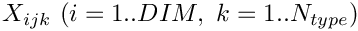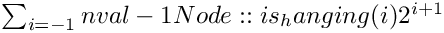where A is the ratio of the aziumuthal to the radial buckling amplitude (A=-1/N for statically buckling rings) and epsilon is the buckling amplitude. Scale R_0 is adjusted to ensure conservation of (computational) volume/area. This is implemented by a pseudo-elasticity approach: The governing equation for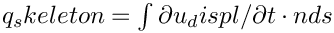is: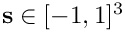The pointer to the reference pressure needs to be set with reference_pressure_pt().

Definition at line 582 of file pseudo_buckling_ring.h.

## ◆ PseudoBucklingRingElement() [1/3]

 oomph::PseudoBucklingRingElement::PseudoBucklingRingElement ( const double & eps_buckl, const double & ampl_ratio, const unsigned n_buckl, const double & r_0, const double & T, TimeStepper * time_stepper_pt )
inline

Constructor: Build pseudo buckling ring from doubles that describe the geometry.

Definition at line 614 of file pseudo_buckling_ring.h.

## ◆ PseudoBucklingRingElement() [2/3]

 oomph::PseudoBucklingRingElement::PseudoBucklingRingElement ( const double & eps_buckl, const double & HoR, const unsigned & n_buckl, const unsigned & imode, TimeStepper * time_stepper_pt )
inline

Constructor: Pass buckling amplitude, h/R, buckling wavenumbe and pointer to global timestepper. Other parameters get set up to represent oscillating ring with mode imode (1 or 2). All geometric data is pinned by default.

Definition at line 648 of file pseudo_buckling_ring.h.

## ◆ PseudoBucklingRingElement() [3/3]

 oomph::PseudoBucklingRingElement::PseudoBucklingRingElement ( const PseudoBucklingRingElement & dummy )
delete

Broken copy constructor.

## ◆ ~PseudoBucklingRingElement()

 virtual oomph::PseudoBucklingRingElement::~PseudoBucklingRingElement ( )
inlinevirtual

Destructor: Kill internal data and set to NULL.

Definition at line 682 of file pseudo_buckling_ring.h.

References oomph::PseudoBucklingRing::Must_clean_up.

## ◆ geometric_local_eqn()

 int oomph::PseudoBucklingRingElement::geometric_local_eqn ( )
inlineprivate

Return the local equation number of the internal geometric variable.

Definition at line 599 of file pseudo_buckling_ring.h.

Referenced by get_residuals_generic().

## ◆ get_jacobian()

 virtual void oomph::PseudoBucklingRingElement::get_jacobian ( Vector< double > & residuals, DenseMatrix< double > & jacobian )
inlinevirtual

Compute element residual Vector and element Jacobian matrix (wrapper)

Reimplemented from oomph::GeneralisedElement.

Definition at line 704 of file pseudo_buckling_ring.h.

References get_residuals_generic().

## ◆ get_residuals()

 virtual void oomph::PseudoBucklingRingElement::get_residuals ( Vector< double > & residuals )
inlinevirtual

Compute element residual Vector (wrapper)

Reimplemented from oomph::GeneralisedElement.

Definition at line 693 of file pseudo_buckling_ring.h.

References get_residuals_generic().

## ◆ get_residuals_generic()

 virtual void oomph::PseudoBucklingRingElement::get_residuals_generic ( Vector< double > & residuals, DenseMatrix< double > & jacobian, unsigned flag )
inlineprotectedvirtual

Compute element residual Vector (only if flag=0) and also element Jacobian matrix (if flag=1)

Definition at line 745 of file pseudo_buckling_ring.h.

Referenced by get_jacobian(), and get_residuals().

## ◆ operator=()

 void oomph::PseudoBucklingRingElement::operator= ( const PseudoBucklingRingElement & )
delete

Broken assignment operator.

## ◆ reference_pressure()

 double oomph::PseudoBucklingRingElement::reference_pressure ( ) const
inline

Return the reference pressure.

Definition at line 718 of file pseudo_buckling_ring.h.

References External_reference_pressure_pt, and oomph::Data::value().

Referenced by get_residuals_generic().

## ◆ reference_pressure_local_eqn()

 int oomph::PseudoBucklingRingElement::reference_pressure_local_eqn ( )
inlineprivate

Return the local equation number of the reference pressure variable.

Definition at line 605 of file pseudo_buckling_ring.h.

Referenced by get_residuals_generic().

## ◆ reference_pressure_pt()

 Data *const & oomph::PseudoBucklingRingElement::reference_pressure_pt ( ) const
inline

Pointer to pressure data that is used as reference pressure.

Definition at line 712 of file pseudo_buckling_ring.h.

## ◆ set_reference_pressure_pt()

 void oomph::PseudoBucklingRingElement::set_reference_pressure_pt ( Data *const & data_pt )
inline

Set the pressure data that is used as reference pressure.

Definition at line 732 of file pseudo_buckling_ring.h.

## ◆ External_reference_pressure_index

 unsigned oomph::PseudoBucklingRingElement::External_reference_pressure_index
private

The Data object that represents the reference pressure is stored at the location indexed by this integer in the external data storage.

Definition at line 592 of file pseudo_buckling_ring.h.

Referenced by reference_pressure_local_eqn(), and set_reference_pressure_pt().

## ◆ External_reference_pressure_pt

 Data* oomph::PseudoBucklingRingElement::External_reference_pressure_pt
private

Pointer to the data object that represents the external reference pressure.

Definition at line 596 of file pseudo_buckling_ring.h.

Referenced by reference_pressure(), and set_reference_pressure_pt().

## ◆ Internal_geometric_variable_index

 unsigned oomph::PseudoBucklingRingElement::Internal_geometric_variable_index
private

Index of the value stored in the single geometric object that has become an unknown.

Definition at line 588 of file pseudo_buckling_ring.h.

Referenced by geometric_local_eqn(), and PseudoBucklingRingElement().

The documentation for this class was generated from the following file: